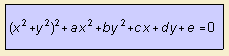# bicircular quartic

## quartic

last updated: 2004-12-04The bicircular quartic is a bicircular algebraic curve that is a quartic.
The curve is the cyclic of a conic.

When the conic has the Cartesian equation x2 / l + y2 / m = 1, the fixed point is (n, o), and the power of inversion is p, then l, m, n, o and p can be written as function of a, b, c, d and e 1).

p=0
When the power of inversion (p) is equal zero, the curve is called rational: a bicircular rational quartic.
This curve is the pedal of the circle with respect to one of its points.

We know the following species:

p<>0
For p <> 0, the the following curves can be distinguished:

The bicircular quartic can also be written in a tripolar equation:

f r1 + g r2 + h r3 = 0.

notes

1) As follows:
a = - 2 (2l+n2+o2-p)
b = - 2 (2m+n2+o2-p)
c = 4ln
d = 4mo
e = (n2+o2-p)2 - 4 (ln2+mo2)